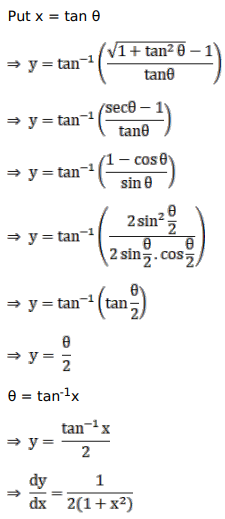# Solve this following

Question:

Mark $(\sqrt{)}$ against the correct answer in the following:

If $y=\tan ^{-1}\left\{\frac{\sqrt{1+x^{2}-1}}{x}\right\}$ then $\frac{d y}{d x}=?$

A. $\frac{1}{\left(1+x^{2}\right)}$

B. $\frac{2}{\left(1+x^{2}\right)}$

C. $\frac{1}{2\left(1+x^{2}\right)}$

D. none of these

Solution: